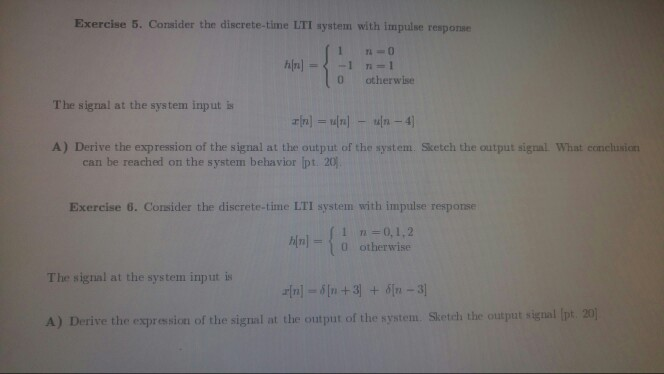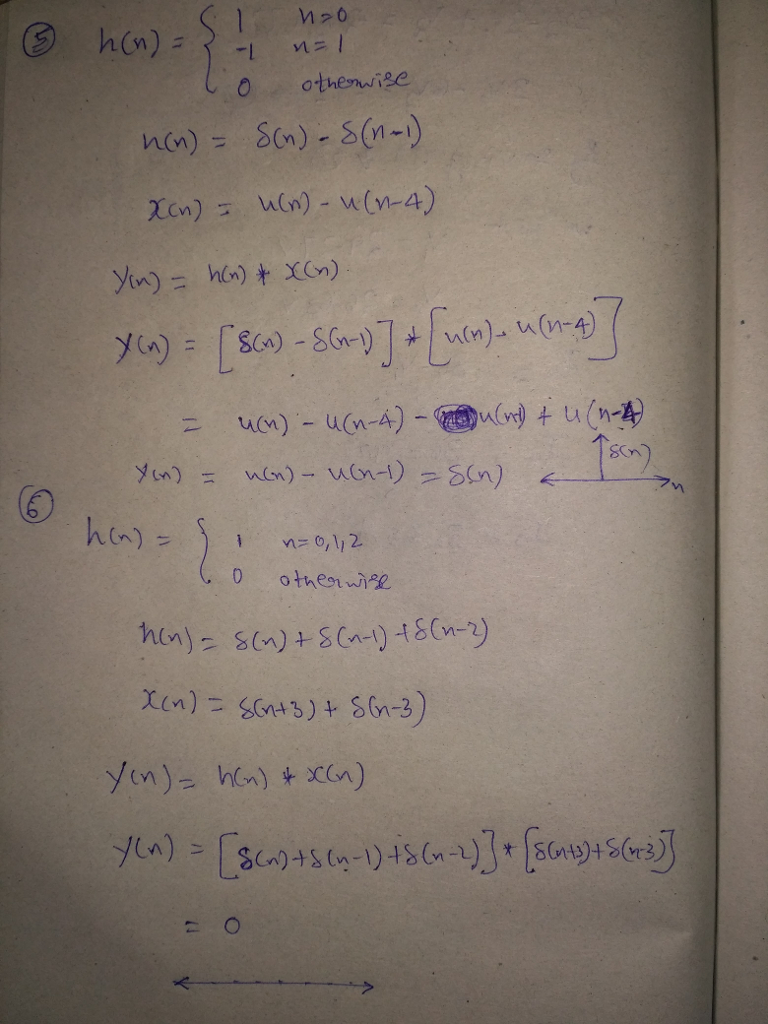# Homework Solution: Consider the discrete-time LTI system with impulse response h[n] = {1 n = 0 – 1 n = 1 0 otherwise The signal at the system input is…Consider the discrete-time LTI system with impulse response h[n] = {1 n = 0 - 1 n = 1 0 otherwise The signal at the system input is x[n] = u[n] - u[n - 4] A) Derive the expression of the signal at the output of the system. Sketch the output signal What conclusion can be reached on the system behaviour. Consider the discrete-time LTI system with impulse response h[n] = {1 n = 0, 1, 2 0 otherwise The signal at the system input is x[n] = delta[n + 3] + delta[n - 3] A) Derive the expression of the signal at the output of the system. Sketch the output signal.